# Old-school debugging

Tags

### Buggy Bug, the Bugster

- - CUT SCREEN HERE - -

Work out how many grams of dilithium crystal it'll take to ship some quonks from Blutol to Skintal.

• Each quonk takes 0.1 grams to ship (they're heavy).
• Add 0.4 grams for pirate insurance. It's optional.

Add input data to the end of the URL, like:

• .../shipping.php?quonks=311&insured=n
•
• .../shipping.php?quonks=9311&insured=Y
•
• .../shipping.php?quonks=666&insured=y

Notice that insured can be upper or lowercase. Assume both params are present, and have no errors.

- - CUT SCREEN HERE - -

Here's a solution:

• <?php
• // Get input.
• \$quonks = \$_GET['quonks'];
• \$insured = \$_GET['insured'];
• // Compute shipping cost in crystals.
• \$cost = \$quonks * 0.1 + 0.3;
• if (\$insured == 'y') {
•     \$cost += 0.4;
• }
• ?><!doctype html>
• <html lang="en">
•         <title>Crystals</title>
•         <meta charset="utf-8">
•         <meta name="viewport" content="width=device-width, initial-scale=1, shrink-to-fit=no">
•     <body>
•         <h1>Dilithium Crystals for Order</h1>
•         <p>Quonks: <?= \$quonks ?>
•         <p>Insurance? <?= \$insured ?></p>
•         <p>Cost: <?= \$cost ?></p>
•     </body>
• </html>

When I try it like this...

• ...something.php?quonks=1&insured=Y

... I should get...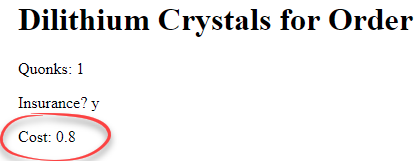But I get...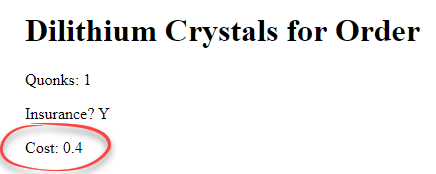You can try it.

How to work out what's wrong?

### What it does versus what it should do

There are two parts to debugging:

• Finding the code where the bug happens.
• Fixing it.

The first one is usually the hardest. You want to work out where in the code the error is, either because your code is wrong, or something is missing.

So, how to find the bug? You compare what the code actually does, with what the code should do.

We know we get the wrong output:The bug is somewhere in the code:

• <?php
• // Get input.
• \$quonks = \$_GET['quonks'];
• \$insured = \$_GET['insured'];
• // Compute shipping cost in crystals.
• \$cost = \$quonks * 0.1 + 0.3;
• if (\$insured == 'y') {
•     \$cost += 0.4;
• }
• ?><!doctype html>
• ...
•     <p>Cost: <?= \$cost ?></p>
• ...
• </html>

The bug could be in the...

• Input code (the GET part). Maybe the code is getting the wrong value.
• Computation part. Maybe the formula is wrong, or something.
• Output code. Maybe the wrong thing is being shown.

How to work out where the problem is?

Let's take the first possibility: the input code is wrong. That would mean that `\$quonks` and/or `\$insured` is not being read correctly.

How to tell? Let's output the variables, just after they're read:

1. <?php
2. // Get input.
3. \$quonks = \$_GET['quonks'];
4. \$insured = \$_GET['insured'];
5. print "<p>
6.     <pre>After GET: quonks: *\$quonks*, insured: *\$insured*</pre>
7. </p>";
8. // Compute shipping cost in crystals.
9. ...

Line 3 reads `\$quonks`. Line 4 reads `\$insured`. Lines 5 to 7 output what was just read, so we can compare what the program does, with what we expect.

We'll talk about line 6 in a moment.

The URL...

• ...something.php?quonks=1&insured=Y

... should set `\$quonks` to 1, and `\$insured` to Y. Lines 5 to 7 tells us what actually happened. You can try it.

Here's what we get: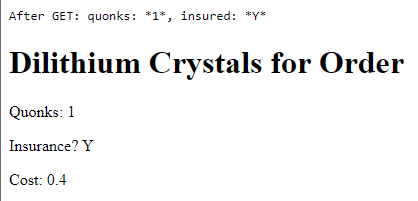Hmm. `\$quonks` and `\$insured` are what we expect. So we can rule out the first bug possibility:

• Input code (the GET part). Maybe the code is getting the wrong value. OK
• Computation part. Maybe the formula is wrong, or something.
• Output code. Maybe the wrong thing is being shown.

Here's the debugging code again:

1. print "<p>
2.     <pre>After GET: quonks: *\$quonks*, insured: *\$insured*</pre>
3. </p>";

Let's break down line 6:

• <pre> After GET: quonks: *\$quonks*, insured: *\$insured* </pre>

`pre` means preformatted. That renders as a fixed space font, that is, it looks like it was typed on a typewriter: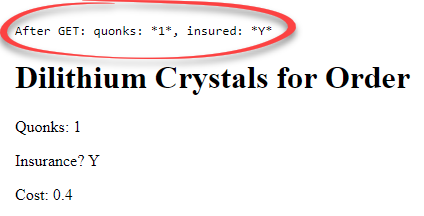In the font you're looking at right now, in this sentence, some characters are easy to confuselike l, and I. In a fixed space font, they look different.

Notice the * before and after each variable:

• <pre>After GET: quonks: *\$quonks*, insured: *\$insured* </pre>

Some errors are caused by extra spaces. They're invisible. Adding * in a fixed space font makes it easy to see extra spaces.

OK, we can rule out the first bug possibility:

• Input code (the GET part). Maybe the code is getting the wrong value. >>OK<<
• Computation part. Maybe the formula is wrong, or something.
• Output code. Maybe the wrong thing is being shown

Let's check the next possibility, that there's an error in the computation.

I'll change the code to...

1. <?php
2. // Get input.
3. \$quonks = \$_GET['quonks'];
4. \$insured = \$_GET['insured'];
5. print "<p>
6.     <pre>After GET: quonks: *\$quonks*, insured: *\$insured*</pre>
7. </p>";
8. // Compute shipping cost in crystals.
9. \$cost = \$quonks * 0.1 + 0.3;
10. print "<p>
11.     <pre>cost: *\$cost*</pre>
12. </p>";...

Lines 10 to 12 are new.

When we try...

• ...something.php?quonks=1&insured=Y

... we expect cost to be 0.4 at line 11. Here's what we get: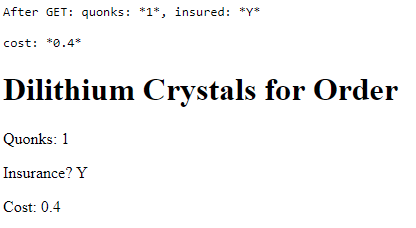Hmm. Looks OK. You can try it.

The computation part doesn't seem to be the problem.

• Input code (the GET part). Maybe the code is getting the wrong value. OK
• Computation part. Maybe the formula is wrong, or something. OK
• Output code. Maybe the wrong thing is being shownWait. You haven't finished the computation yet. There's the `if` statement.

Oh, right. I jumped the gun. Here's some new code.

1. <?php
2. // Get input.
3. \$quonks = \$_GET['quonks'];
4. \$insured = \$_GET['insured'];
5. print "<p>
6.     <pre>After GET: quonks: *\$quonks*, insured: *\$insured*</pre>
7. </p>";
8. // Compute shipping cost in crystals.
9. \$cost = \$quonks * 0.1 + 0.3;
10. print "<p>
11.     <pre>cost: *\$cost*</pre>
12. </p>";
13. if (\$insured == 'y') {
14.     \$cost += 0.4;
15.     print "<p>
16.         <pre>Inside if: cost: *\$cost*</pre>
17.     </p>";
18. }
19. ...

There's a `print` inside the `if`, lines 15 to 17. It outputs "Inside if," so we can tell which code made the output

You can try it

Here's the output: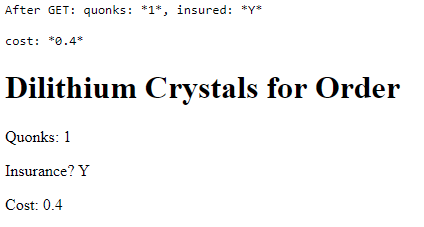Wait, what?! It looks like `<pre>Inside if: cost: *\$cost*</pre>` didn't do anything. Huh. I call shenanigans on the `if`.

Let's add another debugging statement to make sure. It's after the `if`, lines 19 to 21.

1. <?php
2. // Get input.
3. \$quonks = \$_GET['quonks'];
4. \$insured = \$_GET['insured'];
5. print "<p>
6.     <pre>After GET: quonks: *\$quonks*, insured: *\$insured*</pre>
7. </p>";
8. // Compute shipping cost in crystals.
9. \$cost = \$quonks * 0.1 + 0.3;
10. print "<p>
11.     <pre>cost: *\$cost*</pre>
12. </p>";
13. if (\$insured == 'y') {
14.     \$cost += 0.4;
15.     print "<p>
16.         <pre>Inside if: cost: *\$cost*</pre>
17.     </p>";
18. }
19. print "<p>
20.     <pre>After if: *\$cost*</pre>
21. </p>";
22. ?><!doctype html>
23. ...

Here's the output: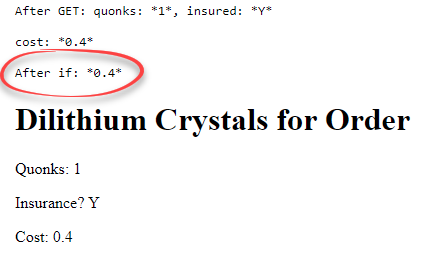OK, that's there. The code isn't running the `if`, suggesting that the buggy line is...

• if (\$insured == 'y') {

So, with the URL...

• ...something.php?quonks=1&insured=Y

... the line...

• if (\$insured == 'y') {

... isn't being run.

At this point, you might work out the issue. But suppose you don't. The `if` looks fine to you.

Hmm. What now?

This line...

• if (\$insured == 'y') {

... should run the code in the `if` when `\$insured == 'y'` is true. We can output the results of that expression, but we have to do some gymnastics to make it work:

1. <?php
2. // Get input.
3. \$quonks = \$_GET['quonks'];
4. \$insured = \$_GET['insured'];
5. print "<p>
6.     <pre>After GET: quonks: *\$quonks*, insured: *\$insured*</pre>
7. </p>";
8. // Compute shipping cost in crystals.
9. \$cost = \$quonks * 0.1 + 0.3;
10. print "<p>
11.     <pre>cost: *\$cost*</pre>
12. </p>";
13. if (\$insured == 'y') { New
14.     \$comparison = 'true';
15. }
16. else {
17.     \$comparison = 'false';
18. }
19. print "<p>
20.     <pre>Before if: \$insured == 'y': *{\$comparison}*</pre>
21. </p>"; / new
22. if (\$insured == 'y') {
23.     \$cost += 0.4;
24.     print "<p>
25.         <pre>Inside if: cost: *\$cost*</pre>
26.     </p>";
27. }
28. print "<p>
29.     <pre>After if: *\$cost*</pre>
30. </p>";
31. ?><!doctype html>
32. ...

`\$insured == 'y'` is either `true` or `false`. It's a boolean. If you output something that's `false`, you get no output. You can't tell if it's false, or your debugging statement is broken.

Let's convert the boolean into a string. Lines 13 to 18 do that:

• if (\$insured == 'y') { New
•     \$comparison = 'true';
• }
• else {
•     \$comparison = 'false';
• }

We make a new variable, `\$comparison`. It ends up with the string `'true'`, or the string `'false'`.

Lines 19 to 21 show the results:

• print "<p>
•     <pre>Before if: \$insured == 'y': *{\$comparison}*</pre>
• </p>";

Because of the double quotes ("), this will output the value of `\$insured`, not the text "\$insured". That will make it easier to interpret the results.

Here's what we see: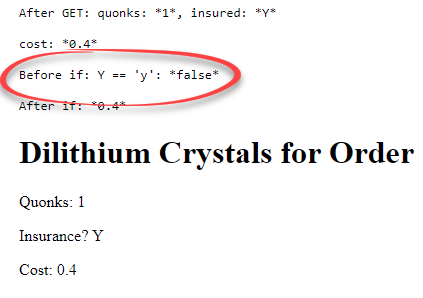You can try it.

Aha! There's the bug! I forgot to convert user input to lowercase, before the `if`.

### Fixing the error

Ain't nothin' to it. Add a line of code:

• <?php
• // Get input.
• \$quonks = \$_GET['quonks'];
• \$insured = \$_GET['insured'];
• \$insured = strtolower(\$insured);
• // Compute shipping cost in crystals.

### Summary

• There are two parts to debugging:
• Finding the code where the bug happens.
• Fixing it.
• You compare what the code does, with what the code should do.
• Output variables with `print`, to see what your program does.

### Up next

Now let's check out a different way of debugging, with PHPStorm, and XDebug. It's better, but has some complex set up.